This is the bipartite network consisting of Twitter users and the URLs they mentioned in their postings. Left nodes represent users and right nodes represent URLs. An edge shows that an URL was mentioned by a user in a tweet.

 Code `Wui` Internal name `munmun_twitterex_ui` Name Twitter user–item Data source http://www.public.asu.edu/~mdechoud/datasets.html Availability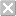Dataset is not available for download Consistency checkDataset passed all tests Category Interaction network Dataset timestamp 2010 Node meaning User, URL Edge meaning Mention Network formatBipartite, undirected Edge typeUnweighted, multiple edges Temporal dataEdges are annotated with timestamps Snapshot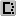Is a snapshot and likely to not contain all data

## Statistics

 Size n = 9,374,206 Left size n1 = 244,537 Right size n2 = 9,129,669 Volume m = 12,656,613 Unique edge count m̿ = 10,214,177 Wedge count s = 708,995,210 Claw count z = 1,155,590,474,676 Cross count x = 5,291,997,635,686,715 Square count q = 53,711,135 4-Tour count T4 = 3,286,104,890 Maximum degree dmax = 24,106 Maximum left degree d1max = 855 Maximum right degree d2max = 24,106 Average degree d = 2.700 31 Average left degree d1 = 51.757 5 Average right degree d2 = 1.386 32 Fill p = 4.575 13 × 10−6 Average edge multiplicity m̃ = 1.239 12 Size of LCC N = 7,488,524 Diameter δ = 30 50-Percentile effective diameter δ0.5 = 7.524 28 90-Percentile effective diameter δ0.9 = 9.761 17 Median distance δM = 8 Mean distance δm = 8.134 08 Gini coefficient G = 0.623 814 Balanced inequality ratio P = 0.263 288 Left balanced inequality ratio P1 = 0.285 079 Right balanced inequality ratio P2 = 0.418 375 Relative edge distribution entropy Her = 0.906 108 Power law exponent γ = 8.945 62 Tail power law exponent γt = 3.261 00 Degree assortativity ρ = −0.042 714 3 Degree assortativity p-value pρ = 0.000 00 Spectral norm α = 1,713.76 Spectral separation |λ1[A] / λ2[A]| = 1.750 05 Controllability C = 8,893,258 Relative controllability Cr = 0.948 695

## Plots

### Degree distribution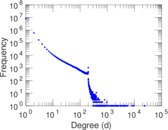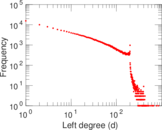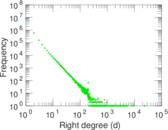### Cumulative degree distribution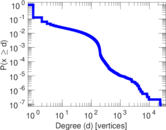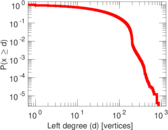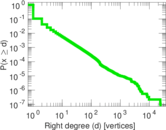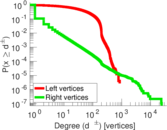### Lorenz curve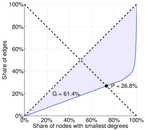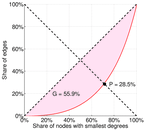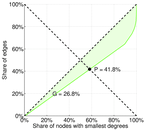### Spectral distribution of the adjacency matrix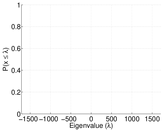### Spectral distribution of the normalized adjacency matrix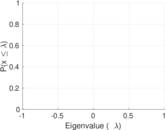### Spectral distribution of the Laplacian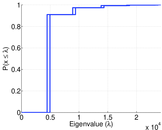### Spectral graph drawing based on the adjacency matrix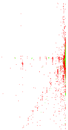### Spectral graph drawing based on the Laplacian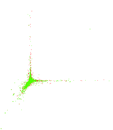### Spectral graph drawing based on the normalized adjacency matrix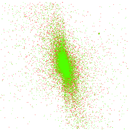### Degree assortativity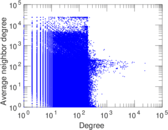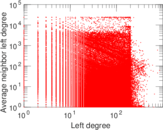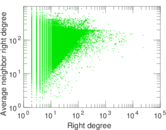### Hop distribution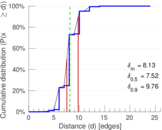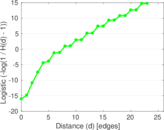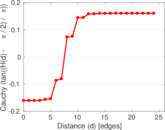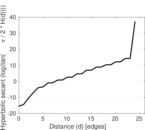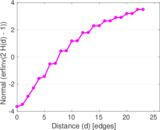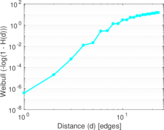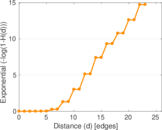### Edge weight/multiplicity distribution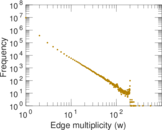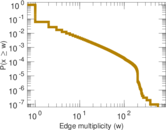### Temporal distribution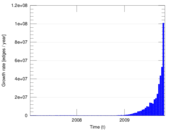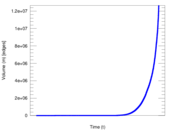### Diameter/density evolution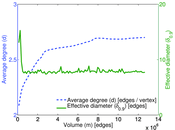### Inter-event distribution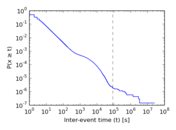### Node-level inter-event distribution